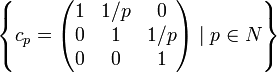# There exist infinite nilpotent groups in which every automorphism is inner

## Statement

It is possible to have an infinite nilpotent group in which every automorphism is inner: an infinite nilpotent group$G$ that is also a group in which every automorphism is inner. In other words,$G$ is infinite and nilpotent and every automorphism of$G$ is an inner automorphism, or equivalently, the outer automorphism group of$G$ is the trivial group.

## Related facts

### Opposite facts

Group type Opposite fact Proved in ... Implication in our language
group of prime power order, hence also finite nilpotent group Group of prime power order is either trivial or of prime order or has outer automorphism class of same prime order Nichtabelsche $p$-Gruppen besitzen äussere $p$-Automorphismen by Wolfgang Gaschütz, Journal of Algebra, ISSN 00218693, Volume 4, Page 1 - 2(Year 1966): More info This shows that with the exception of the trivial group and cyclic group:Z2, every group of prime power order has a nontrivial outer automorphism. To see this, note that if the group is of prime order$p$, the outer automorphism group has order$p - 1$ and is this nontrivial for$p > 2$. In other cases, the outer automorphism group has a subgroup of order$p$, so it is nontrivial.
finite nilpotent group Finite nilpotent and every automorphism is inner implies trivial or cyclic of order two (corollary of previous result) Follows from preceding result and the fact that automorphism group of finite nilpotent group is direct product of automorphism groups of Sylow subgroups.
finitely generated nilpotent group Finitely generated nilpotent and every automorphism is inner implies trivial or cyclic of order two The existence of outer automorphism groups of some groups. I by R. Ree, Proceedings of the American Mathematical Society, Volume 7,Number 6, Page 962 - 964(Year 1956): More info
The existence of outer automorphism groups of some groups. II by R. Ree, Proceedings of the American Mathematical Society, Volume 9,Number 1, Page 105 - 109(Year 1958): More info
Any finitely generated nilpotent group other than the trivial group and cyclic group:Z2 has a nontrivial outer automorphism group.
nilpotent p-group nilpotent p-group and every automorphism is inner implies trivial or cyclic or order two A nilpotent p-group possesses an outer automorphism by A. E. Zalesskii, Dokl. Akad. Nauk SSSR, Volume 196,Number 4, Page 751 - 754(Year 1971): More info Any nilpotent p-group other than the trivial group and cyclic group:Z2 has a nontrivial outer automorphism group.
periodic nilpotent group periodic nilpotent and every automorphism is inner implies trivial or cyclic of order two (follows from preceding result) Any periodic nilpotent group other than the trivial group and cyclic group:Z has a nontrivial outer automorphism group.

## Proof

The proof here is taken directly from the paper that provides the original proof. The group that we construct, called the Zalesskii group, is a subgroup of unitriangular matrix group:UT(3,Q), hence it is a group of nilpotency class two.

### Construction of the group

Further information: Zalesskii group

Step no. Step detail
1 Partition the set of all prime numbers into three pairwise disjoint subsets$L,M,N$ where$2 \in N$, all three of the subsets being infinite.
2 Let$A$ be the set$\left \{ a_p = \begin{pmatrix} 1 & 1/p & 1/p^2 \\ 0 & 1 & 0 \\ 0 & 0 & 1 \\\end{pmatrix} \mid p \in L \right \}$
3 Let$B$ be the set$\left \{ b_p = \begin{pmatrix} 1 & 0 & 1/p^2 \\ 0 & 1 & 1/p \\ 0 & 0 & 1 \\\end{pmatrix} \mid p \in M \right \}$
4 Let$C$ be the set$\left \{ c_p = \begin{pmatrix} 1 & 1/p & 0 \\ 0 & 1 & 1/p \\ 0 & 0 & 1 \\\end{pmatrix} \mid p \in N \right \}$
5 The group$G$ we are interested in is the subgroup$G = \langle A \cup B \cup C \rangle$ inside$UT(3,\mathbb{Q})$, the group of$3 \times 3$ upper-triangular matrices with 1s on the diagonal and rational entries.

### Proof that it works

Step no. Assertion/construction Facts used Given data used Previous steps used Explanation
1 For any subgroup$S$ of$\mathbb{Q}$, the only automorphisms of$S$ are the multiplication maps by$\lambda$ where$\lambda \in \mathbb{Q}, \lambda S = S$. Fact (2)
2 Denote by$\mathbb{Q}_0$ the subgroup of$\mathbb{Q}$ that is the group of rational numbers with square-free denominators. Then, for any positive integer$m$, the subgroup$m\mathbb{Q}_0$ has index$m$ in$\mathbb{Q}_0$. PLACEHOLDER FOR INFORMATION TO BE FILLED IN: [SHOW MORE]
3 The center$Z(G)$ of$G$ is isomorphic to$\mathbb{Q}_0$ and comprises matrices of the form$\begin{pmatrix} 1 & 0 & \gamma \\ 0 & 1 & 0 \\ 0 & 0 & 1 \\\end{pmatrix}$, with$\gamma \in \mathbb{Q}_0$. PLACEHOLDER FOR INFORMATION TO BE FILLED IN: [SHOW MORE]
4 The quotient group$G/Z(G)$ has only two automorphisms: the identity map and the inverse map. Fact (2) PLACEHOLDER FOR INFORMATION TO BE FILLED IN: [SHOW MORE]
5 Every automorphism of$G$ induces the identity map on$Z(G)$ and on$G/Z(G)$ PLACEHOLDER FOR INFORMATION TO BE FILLED IN: [SHOW MORE]
6 Every automorphism of$G$ is inner. PLACEHOLDER FOR INFORMATION TO BE FILLED IN: [SHOW MORE]